# MATLAB Simulink - Sine Wave

In this chapter we will integrate and differentiate sine wave by using the derivative and integrator blocks.

Open blank model and Simulink library as shown below −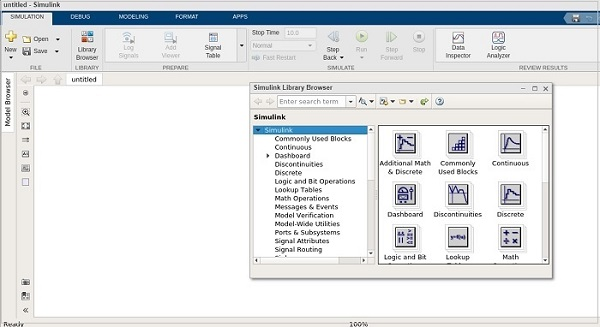Let us pick the sine wave from sources library and scope block from sinks library.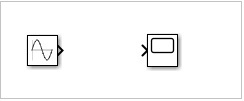We would like to add the derivative and integrator block from continuous library as shown below −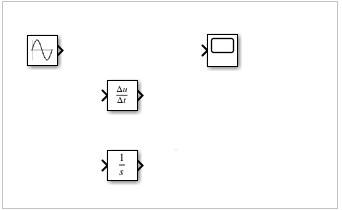We would need 3 input ports for scope block as the sine wave, derivative and integrator block will be connected to it.

Right click on the scope block and change the inputs from 1 to 3 as shown below −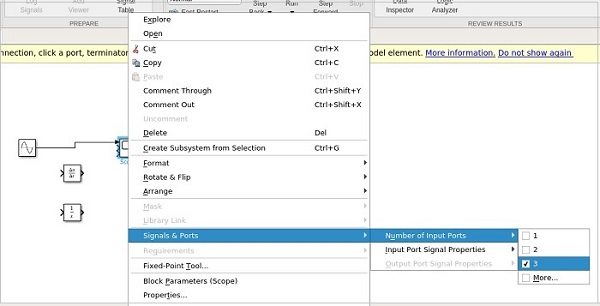Connect the lines as shown below −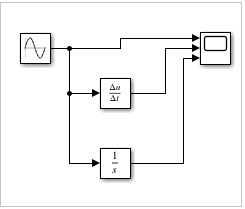Now, run the model to see the display.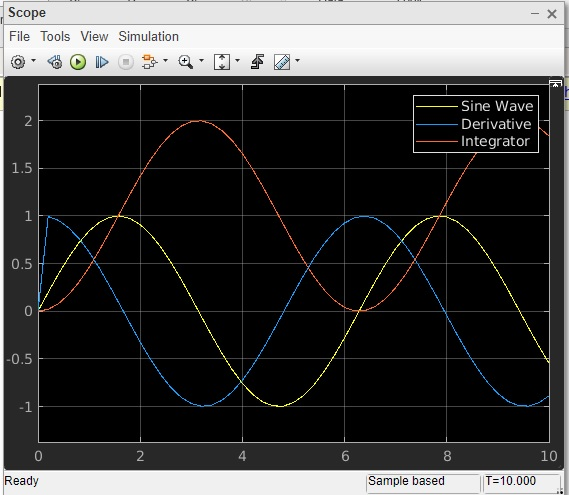So, we have three signals sine wave, derivative and integrator.